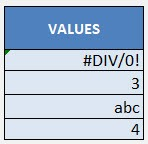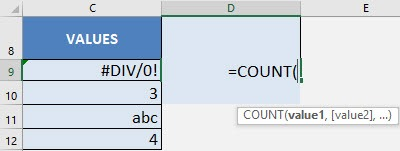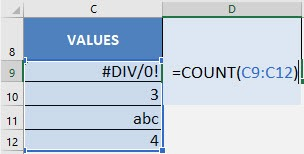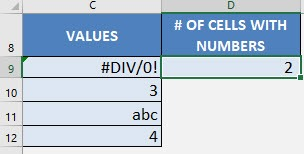What does it do?

Counts the number of cells that contain numbers

Formula breakdown:

=COUNT(value1, [value2]…)

What it means:

=COUNT(range of cells to check, [additional cells to include in the check]…)

Ever had a column of data and wanted to check if all of the values contain valid numbers?

It would be cumbersome to count and check them one by one, especially if you had hundreds of entries!

Imagine we have the following data, we see an error, a text and a couple of numbers:Thankfully there is an easy way to count how many of these cells contain valid numbers using the Excel’s COUNT formula.

I explain how you can do this below:STEP 1: We need to enter the COUNT function in a blank cell:

## =COUNT(STEP 2: The COUNT arguments:

## value

What is the value / range of values that you want to check?

## =COUNT(C9:C12)You now have your count of valid numbers!Count Formula in Excel